# Estimating a Single Sprinkler Discharge

This calculator estimates the sprinkler water application rate by setting up a simple home experiment. In order to replicate the experiment, you will need two sprinklers of the same type, and 4 cans.

Arrange the four cans between two sprinklers as shown in the figure below. Operate the sprinkler for 15 minutes or more and measure the depth of water collected in each can. Enter the measured depths in the following boxes. Learn more about the units used on this page.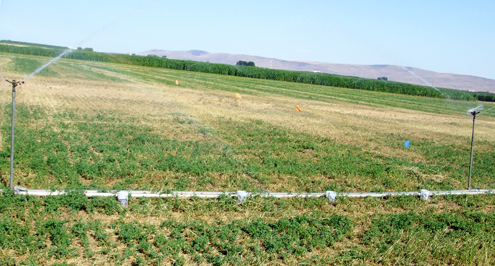## Sprinkler Flow Rate

Can #1 Water Depth:

Can #2 Water Depth:

Can #3 Water Depth:

Can #4 Water Depth:

Sprinkler Operation Time:

Sprinkler Application Rate:

## The Equations

This calculators use these formulas to determine the Sprinkler Flow Rate.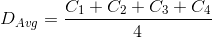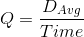Where: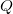= Sprinkler Flow Rate (in/hr)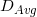= Average depth of the catch cans (in)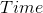= Sprinkler Operation Time (min)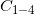= Catch Water Depth (in)

WSU Prosser - IAREC, 24106 N Bunn Rd, Prosser WA 99350-8694, 509-786-2226. Contact Us